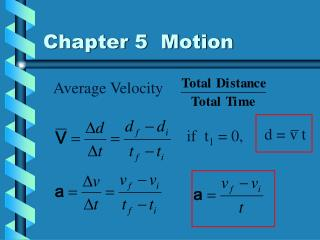DownloadDownload PresentationChapter 5 Motion

# Chapter 5 Motion

Télécharger la présentation## Chapter 5 Motion

- - - - - - - - - - - - - - - - - - - - - - - - - - - E N D - - - - - - - - - - - - - - - - - - - - - - - - - - -
##### Presentation Transcript

1. Chapter 5 Motion d = v t Average Velocity if t1 = 0,

2. Chapter 5 Motion Solve for vf

3. Chapter 5 Motion Solve both equations for t

4. Chapter 5 Motion Set equal to each other

5. Chapter 5 Motion Multiply across

6. Chapter 5 Motion d = v t

7. Chapter 5 Motion • A car is traveling at 65 km/hr. The brakes • are applied and the car stops in 3 seconds. • What is the car’s acceleration? • How far does it travel? vi = 65 km/hr t = 3 s vf = 0 vi = 65 km/hr = 18m/s

8. Chapter 5 Motion • A car is traveling at 65 km/hr. The brakes • are applied and the car stops in 3 seconds. • What is the car’s acceleration? • How far does it travel? vi = 18 m/s t = 3 s vf = 0

9. Chapter 5 Motion • A car is traveling at 65 km/hr. The brakes • are applied and the car stops in 3 seconds. • What is the car’s acceleration? • How far does it travel? =27 m

10. Chapter 5 Motion • An airplane must reach a speed of 55 m/s • before take off. It can accelerate at 12 m/s2. • How long does the runway have to be? • How long will this take? vi = 0 a = 12 m/s2 vf = 55 m/s

11. Chapter 5 Motion • An airplane much reach a speed of 55 m/s • before take off. It can accelerate at 12 m/s2. • How long does the runway have to be? • How long will this take? vi = 0 a = 12 m/s2 vf = 55 m/s

12. Chapter 5 Motion Scott is driving a car at 20 m/s and notices a cow in front of him at a distance of 80 m away. He brakes at a rate of 3 m/s2. What happens to the cow? vi = 20 m/s a = -3 m/s2 vf = 0

13. Chapter 5 Motion The cow survives!!!!

14. Acceleration Due to Gravity ag = -9.8 m/s2 For all free falling bodies on earth, they accelerate downward at 9.8 m/s2.

15. Acceleration Due to Gravity • A ball is thrown upward at 49 m/s. • How long is it in the air? • How high does it go? • How fast is it going when it lands?

16. Acceleration Due to Gravity • How long is it in the air? vi = 49 m/s a = -9.8 m/s2 vf = 0 Total time in air is 10 sec

17. Acceleration Due to Gravity • How high does it go? vi = 49 m/s a = -9.8 m/s2 t = 5 sec

18. Acceleration Due to Gravity • How fast is it going when it lands? vi = 49 m/s a = -9.8 m/s2 t = 10 sec

19. A skier travels from A to B to C to D. What is her average velocity and speed?

20. Stoplight Problem When a traffic light turns green, a waiting car starts off with a constant acceleration of 6 m/s2. At the instant the car begins to accelerate, a truck with constant velocity of 21 m/s passes in the next lane. a. How far will the car travel before it overtakes the truck? b. How fast will the car be traveling when it overtakes the truck? c. Construct a distance vs time graph for the car and the truck on the same graph.

21. Stoplight Problem • a. How far will the car travel before it overtakes • the truck? • Truck d = vt Car d = vit + ½ at2 • d = 21 t d = ½ (6) t2 • 21t = 3t2 • 3t2 – 21t = 0 • 3t(t – 7) = 0 t = 0 s, 7 s

22. Stoplight Problem • b. How fast will the car be traveling when it overtakes • the truck? • d = 21 t = 21(7) = 147m • d = ½ at2 = (1/2)(6)(7)2 = 147m • c. Construct a distance vs time graph for the car and • the truck on the same graph. • Graph y1 = 21t, y2 = 3t2 with the –0 symbol. Under mode select simultaneous with a window of [0,10],[0,150]